# Difference between neighbors in a matrix (Solved)

 Importance: Low ✭
 Author(s): Vadim Lioubimov
 Subject: Combinatorics » Matrices
 Keywords:
 Recomm. for undergrads: yes
 Posted by: Vadim Lioubimov on: May 20th, 2013
 Solved by: Daniel Soltész
Conjecture   Any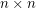matrix with different integer entries has two neighboring entrieswith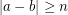.

Only neighbors in the same row or column are considered. Given a matrix, let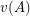be the maximum difference between two neighboring entries of. Given integers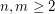, let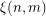be the smallest possible value ofamong all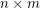matriceswith different integer entries. Thus the conjecture asserts that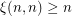.

Consider thematrix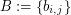defined by. The entries ofisand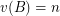. Thus. Consequently the conjecture is equivalent to the assertion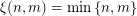.

It can be easily seen that for anymatrixwith different integer entries, there exists a matrix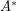with the entriessuch that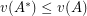. Therefore, in the definition of, we may assume that the entries of each matrix is. Consequently the conjecture reduces to the case when the entries of the matrix is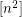.

I have proved the conjecture for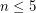. The proof is separate for each of the 4 cases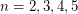and pretty elementary. However, I am not so sure at the moment whether the conjecture is true for all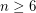. If it turns out to be generally false, it would be an interesting problem to evaluate. I wonder if there are any related results.

## Bibliography

* indicates original appearance(s) of problem.

### Related questions: continued

2. What is the smallest number, denoted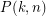, to guarantee that a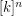-matrixwith the entries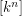has 2 neighboring entriessuch that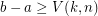and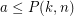? Obviously,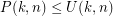, where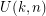is the smallest number such that any subset ofof sizehas at leastneighbors. Due to Theorem 11,is the smallest numbersuch that the initial segment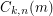of lengthin the simplicial order onhasneighbors. In your proof you used the fact that, which implies by Theorem 11 that. However this bound is not tight. Consider the hamming ball. It is easy to see that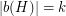and. Thus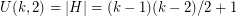. What about higher dimensions?

### Related questions

Dear Daniel, thank you for the very nice proof! Theorem 11 from the lecture notes you refer to suggests some answers to other general questions related to the conjecture (let us now refer to it as Theorem 0). In particular I have the following 2 questions in mind.

1. I wonder how the generalization of Theorem 0 to higher dimensional matrices looks like. More specifically, given integers, what is the smallest value, denoted, ofamong all-matriceswith distinct integer entries? Theorem 0 states that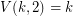. Theorem 11 implies that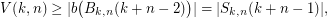where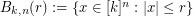and. My feeling is that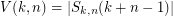. What do you think? Note thatis the number of all ordered partitions ofinto exactlyparts not exceeding.

### bandwidth

Dear Dr. Lioubimov!

At first sight your feeling seemed plausible, but since then i managed to find the more general problem: The graph bandwidth problem. (see wiki page)

At the wikipedia page, you can see that a classical result of Harper is the bandwidth of the hypercube. Well, if your feeling were true, the bandwidth of the cube would be the middle binomial coefficient, which is not true.

We can see this in a different way too, i'll give some heuristics. Take a look at the proof of the 2 dimensional case: We had a single vertex which had a big label (at least n(n-1)/2+n). And we concluded that since it has at least one vertex with "small" label, we found a big difference. If we only have 2 dimensions, this bound is sharp, because the degrees of the vertices are not too big. In higher dimensionan grids there are more neighbours of this vertex with "small" labell. Thus a better lower bound can be obtained.

Sincerely, Daniel Soltész

### Solution

Dear Dr. Lioubimov

Your problem is equivalent to the following: We label the vertces of the nxn grid graph with distinct elements from the set [n^2]. Then there are adjacent vertices such that their labels differ by at least n. I think i found a solution. The key related result is the vertex isoperimetric inequality in the grid. For our problem it is enough to consider the corollary 12 in the following .pdf:

https://www.dpmms.cam.ac.uk/~par31/notes/extcomb.pdf

With parameters: n=2 since we are in a 2 dimensional grid. Let A be the set which have the labels: 1,2,..., n(n-1)/2. Clearly then we can set r=n-1 and with t=1 we obtain that there are at least n neighbors of this set. This finishes our proof since one of these neighbors will have a label of at least n(n-1)/2+n.

Sincerely Daniel Soltész

## Comment viewing options

Select your preferred way to display the comments and click "Save settings" to activate your changes.